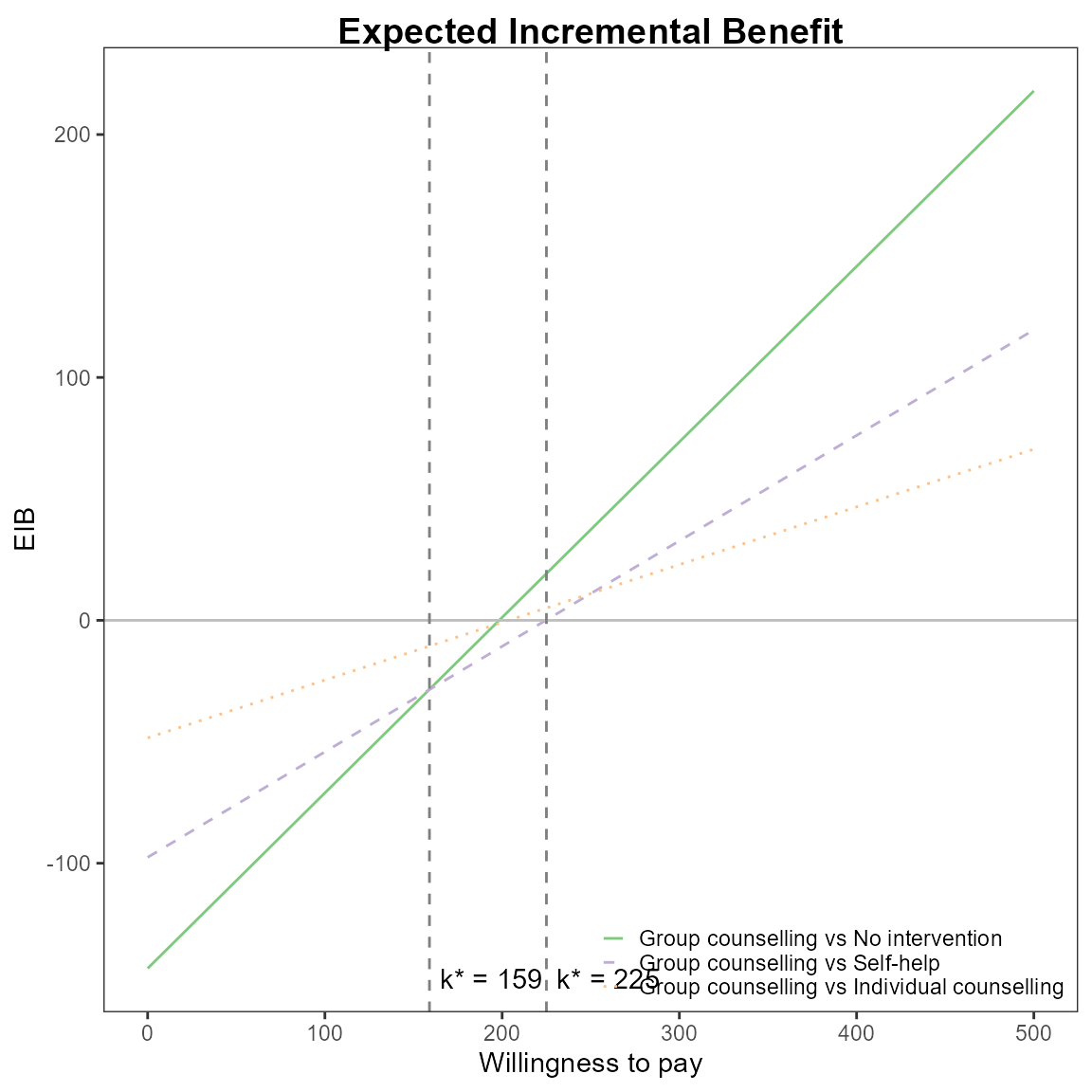## Introduction

The intention of this vignette is to show how to plot different styles of expected incremental benefit (EIB) plots using the BCEA package.

## Two interventions only

This is the simplest case, usually an alternative intervention ($$i=1$$) versus status-quo ($$i=0$$).

The plot is based on the incremental benefit as a function of the willingness to pay $$k$$.

$IB(\theta) = k \Delta_e - \Delta_c$

Using the set of $$S$$ posterior samples, the EIB is approximated by

$\frac{1}{S} \sum_s^S IB(\theta_s)$

where $$\theta_s$$ is the realised configuration of the parameters $$\theta$$ in correspondence of the $$s$$-th simulation.

#### R code

To calculate these in BCEA we use the bcea() function.

data(Vaccine)

he <-
bcea(eff, cost,
ref = 2,
interventions = treats,
Kmax = 50000,
plot = FALSE)

The default EIB plot gives a single diagonal line using base R.

eib.plot(he)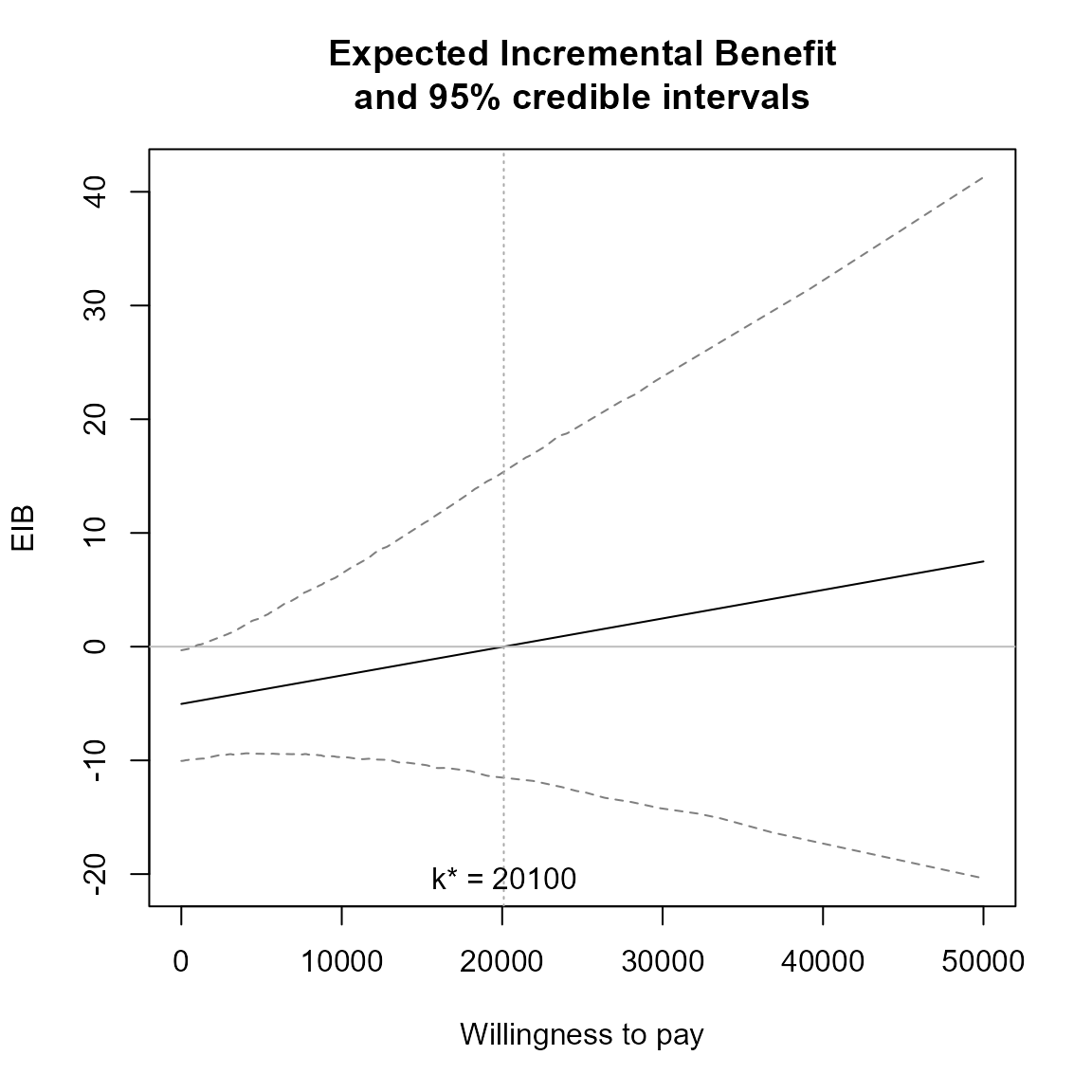The vertical line represents the break-even value corresponding to $$k^*$$ indicating that above that threshold the alternative treatment is more cost-effective than the status-quo.

$k^* = \min\{ k : \mbox{EIB} > 0 \}$

This will be at the point the curve crosses the x-axis.

The plot defaults to base R plotting. Type of plot can be set explicitly using the graph argument.

eib.plot(he, graph = "base")eib.plot(he, graph = "ggplot2")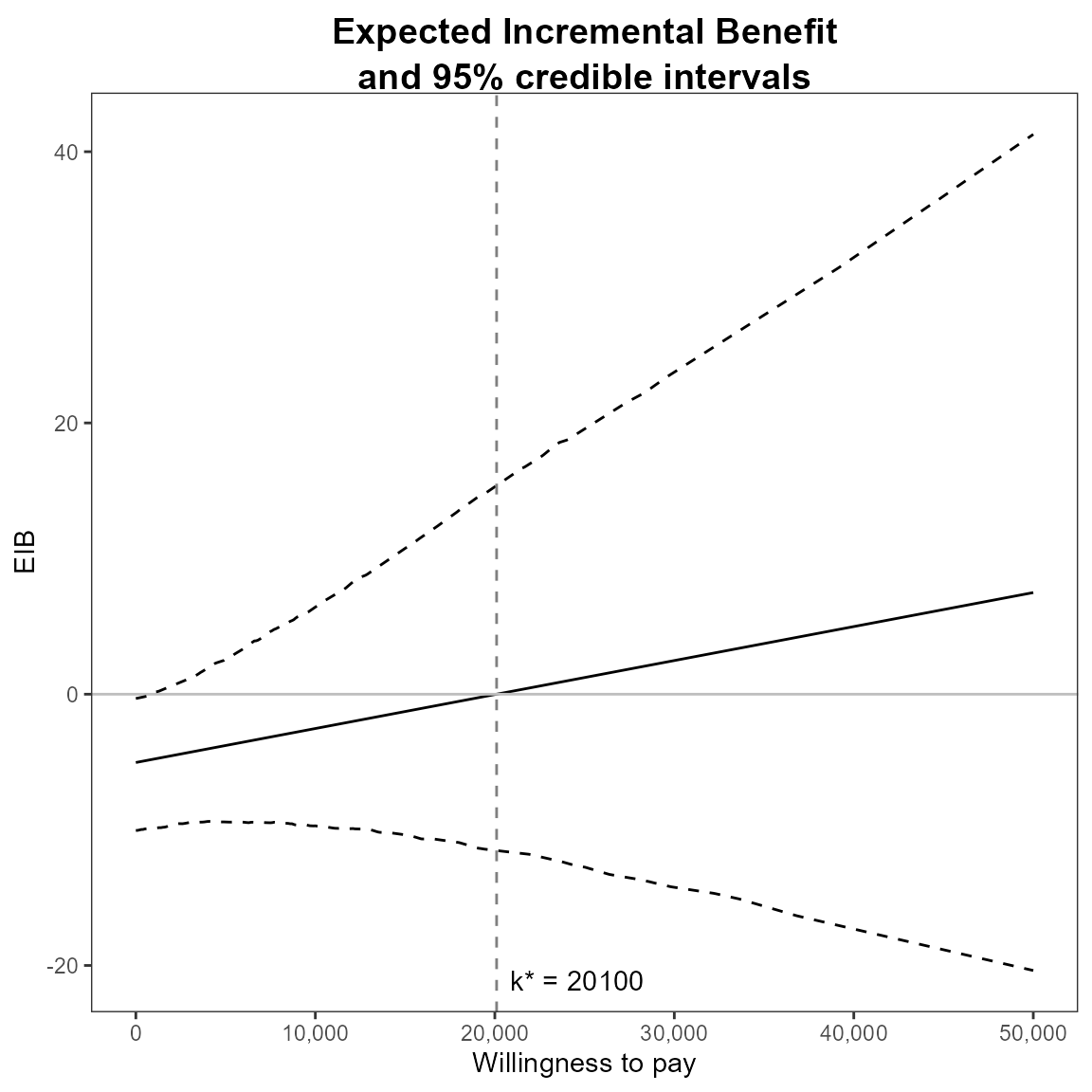# ceac.plot(he, graph = "plotly")

Other plotting arguments can be specified such as title, line colours and theme.

eib.plot(he,
graph = "ggplot2",
main = "my title",
line = list(color = "green"),
theme = theme_dark())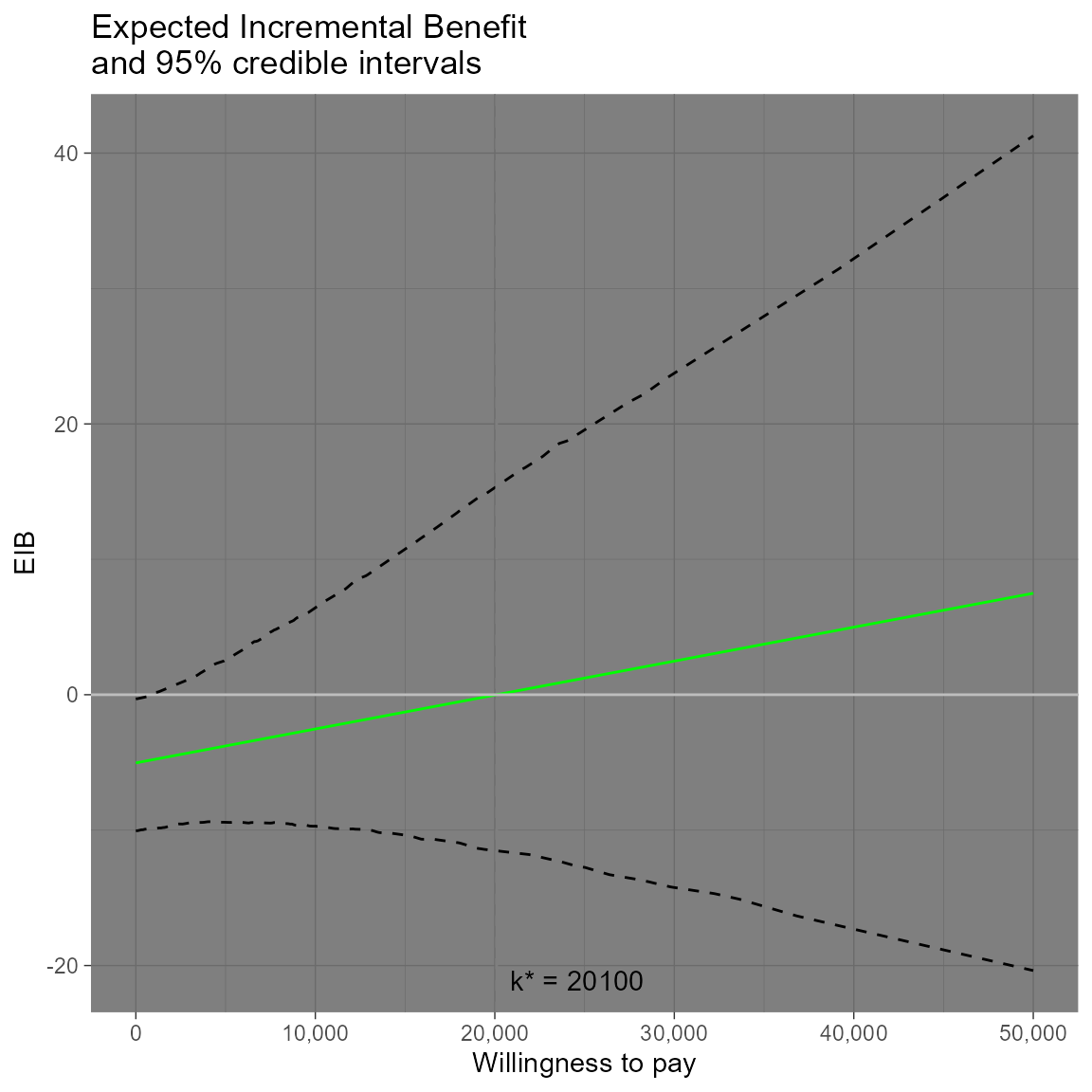Credible interval can also be plotted using the plot.cri logical argument.

eib.plot(he, plot.cri = FALSE)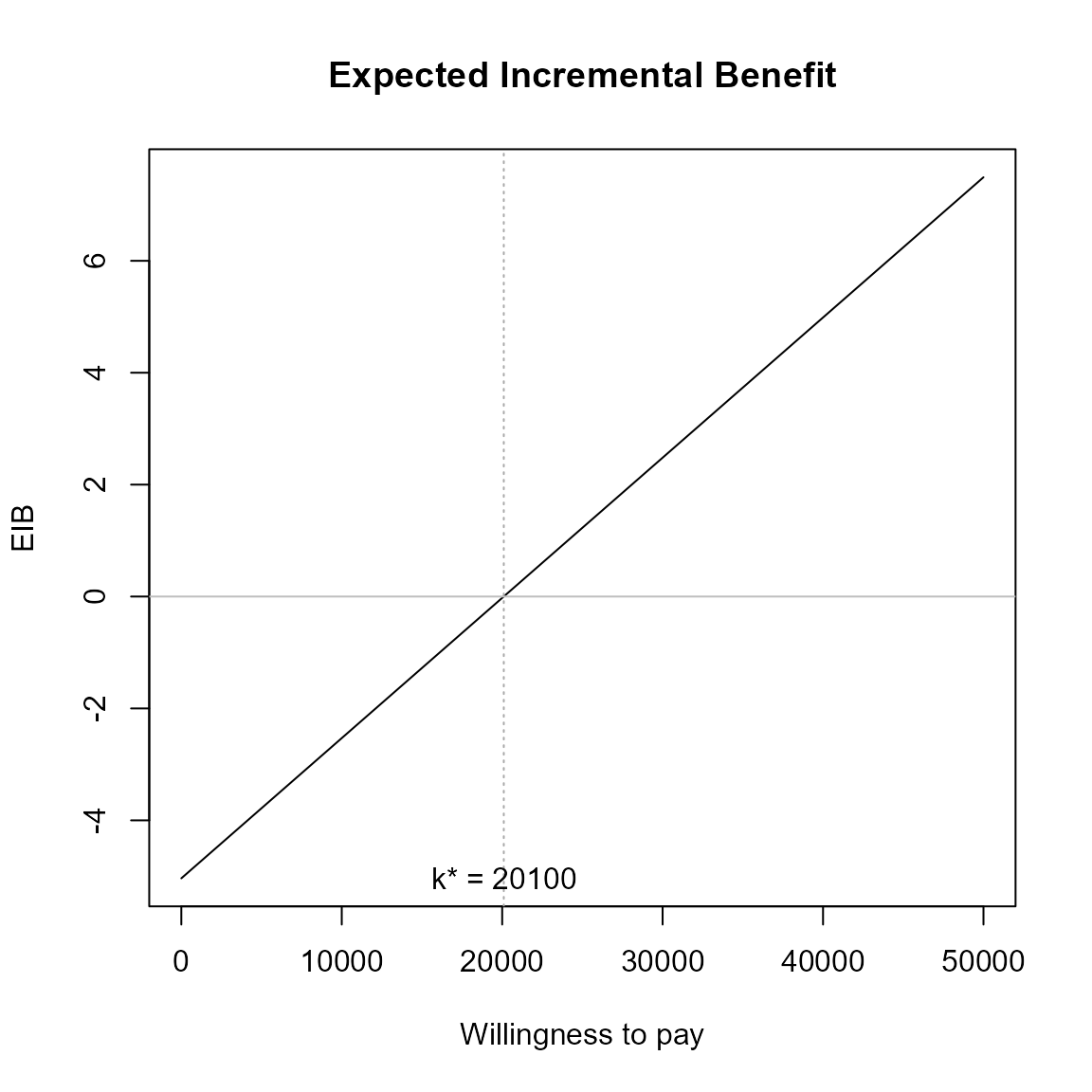## Multiple interventions

This situation is when there are more than two interventions to consider. Incremental values can be obtained either always against a fixed reference intervention, such as status quo, or for all comparisons simultaneously.

The curves are for pair-wise comparisons against a status-quo and the vertical lines and k* annotation is for simultaneous comparisons.

Without loss of generality, if we assume status quo intervention $$i=0$$, then we wish to calculate

$\frac{1}{S} \sum_s^S IB(\theta^{i0}_s) \;\; \mbox{for each} \; i$

The break-even points represent no preference between the two best interventions at $$k$$.

$k^*_i = \min\{ k : \mbox{EIB}(\theta^i) > \mbox{EIB}(\theta^j) \}$

Only the right-most of these will be where the curves cross the x-axis.

#### R code

This is the default plot for eib.plot() so we simply follow the same steps as above with the new data set.

data(Smoking)

treats <- c("No intervention", "Self-help",
"Individual counselling", "Group counselling")
he <- bcea(eff, cost, ref = 4, interventions = treats, Kmax = 500)

eib.plot(he)For example, we can change the main title and the EIB line colours to green.

eib.plot(he,
graph = "base",
main = "my title",
line = list(color = "green"))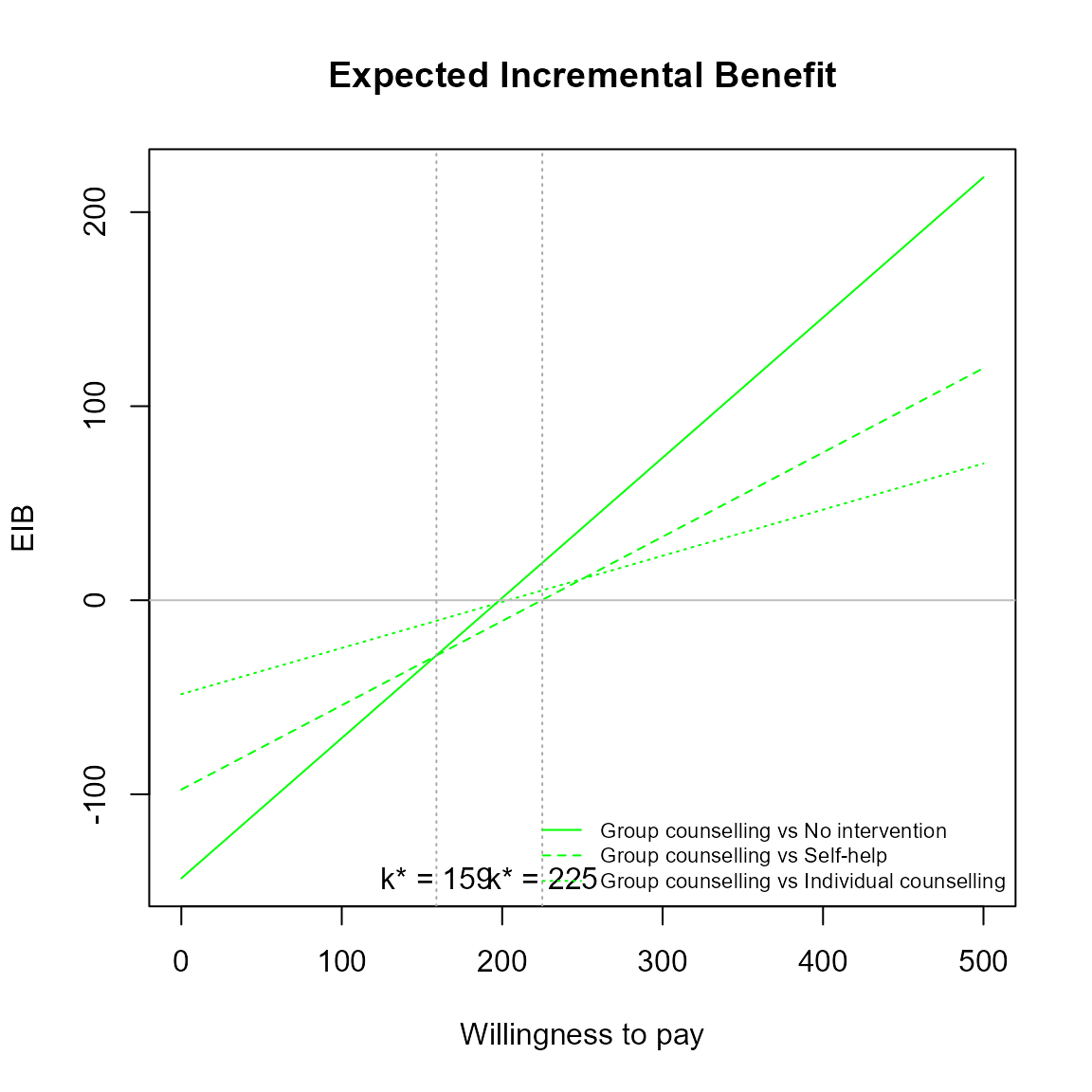eib.plot(he,
graph = "ggplot2",
main = "my title",
line = list(color = "green"))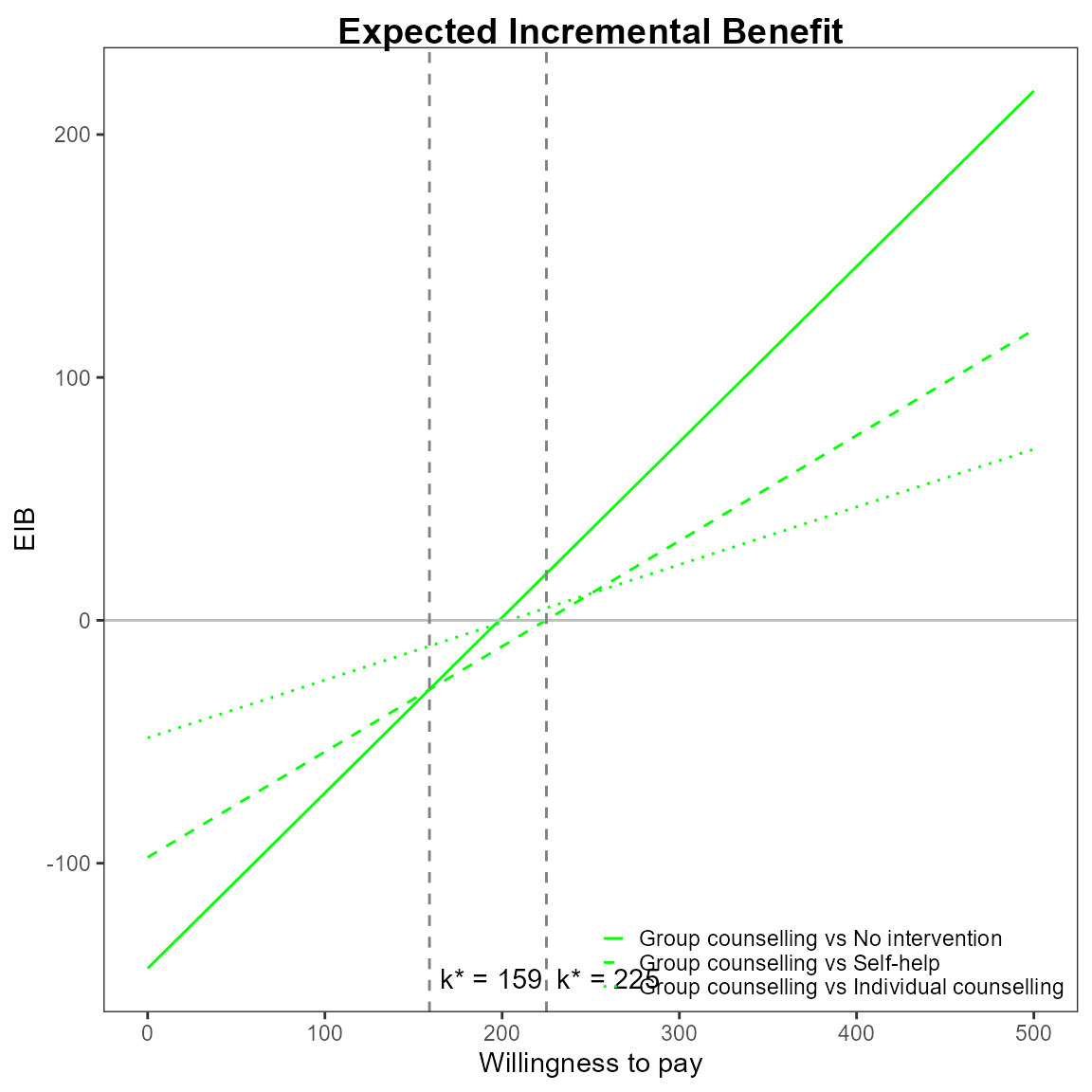Credible interval can also be plotted as before. This isn’t recommended in this case since its hard to understand with so many lines.

eib.plot(he, plot.cri = TRUE)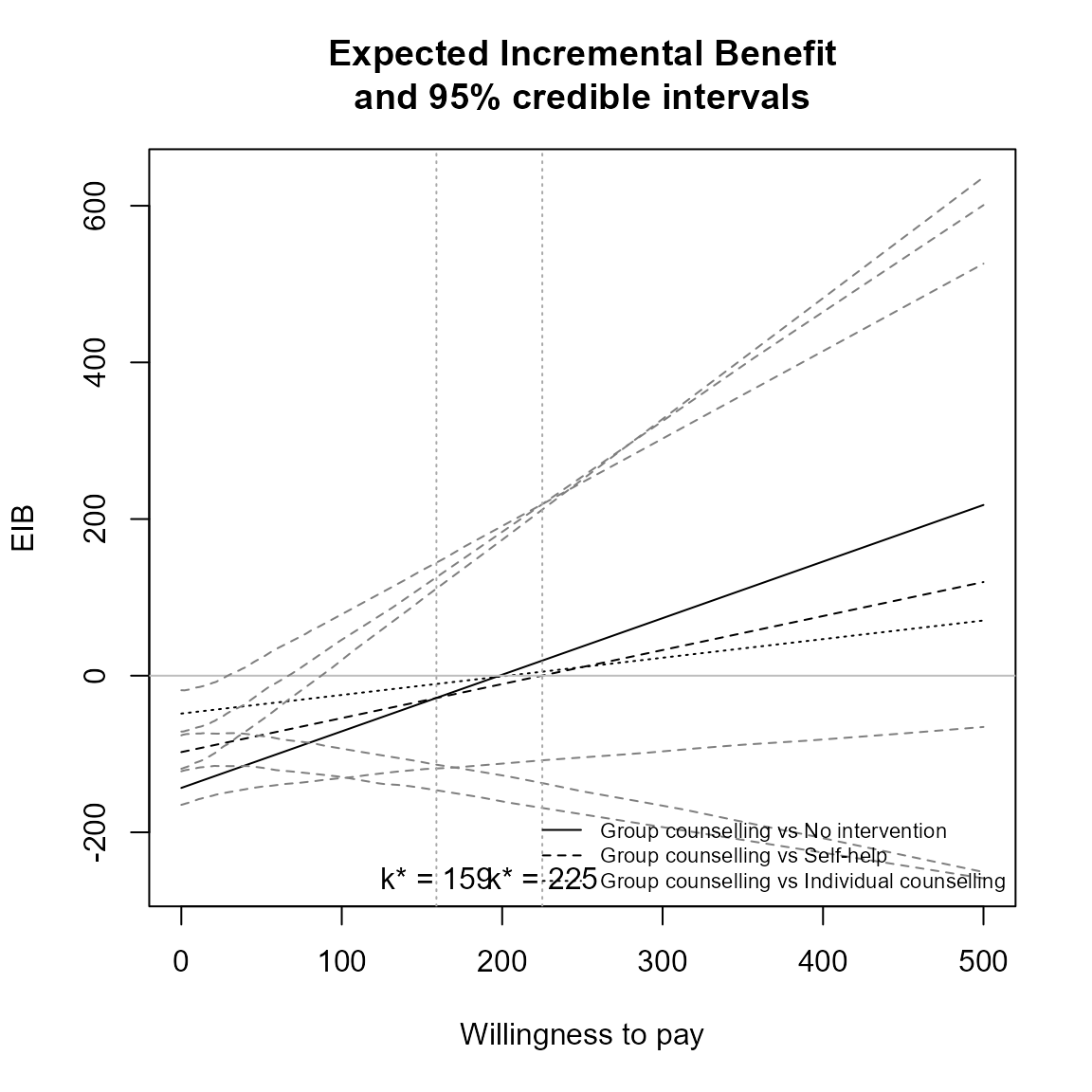##### Repositioning the legend.

For base R,

eib.plot(he, pos = FALSE) # bottom righteib.plot(he, pos = c(0, 0))eib.plot(he, pos = c(0, 1))eib.plot(he, pos = c(1, 0))eib.plot(he, pos = c(1, 1))For ggplot2,

##TODO:
eib.plot(he, graph = "ggplot2", pos = c(0, 0))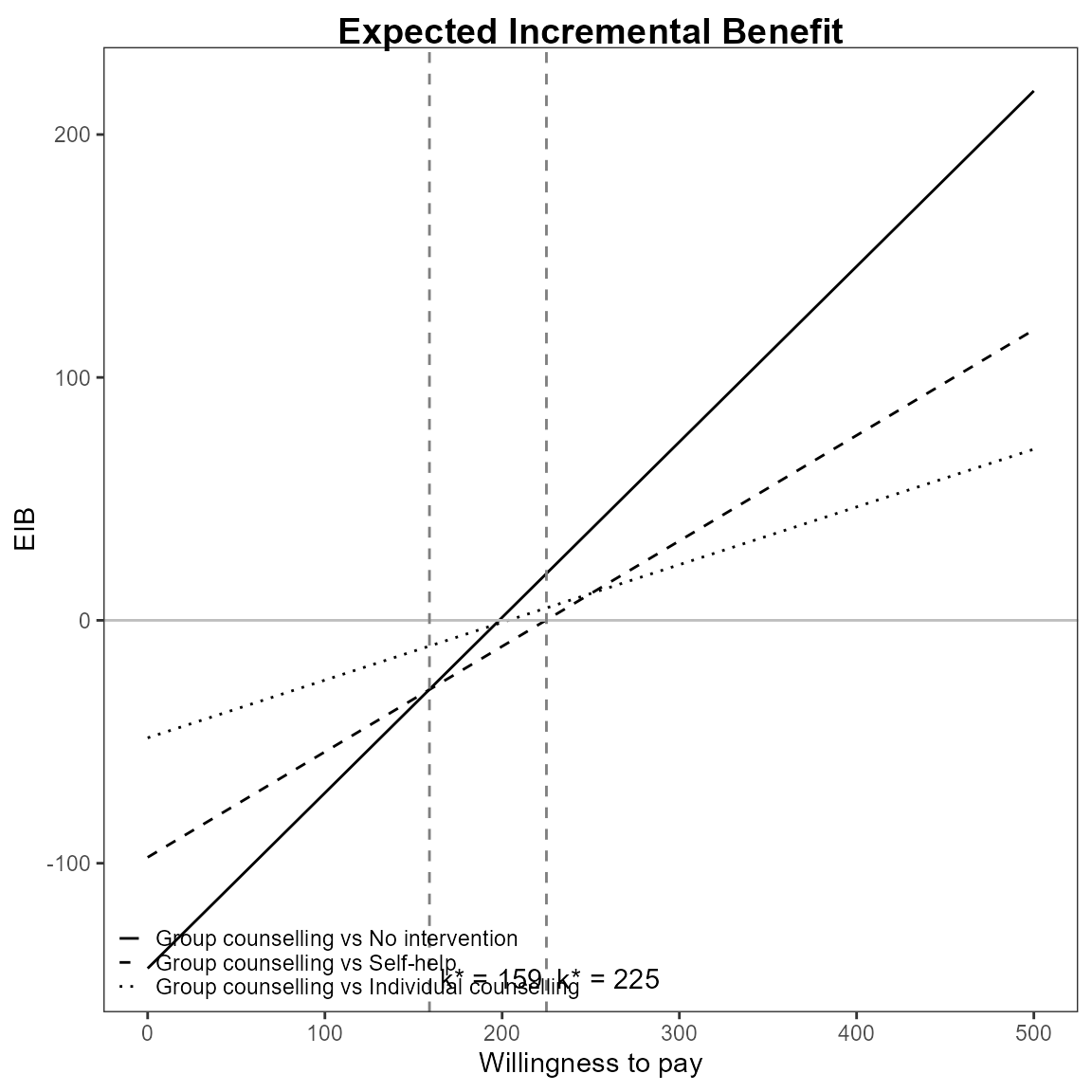eib.plot(he, graph = "ggplot2", pos = c(0, 1))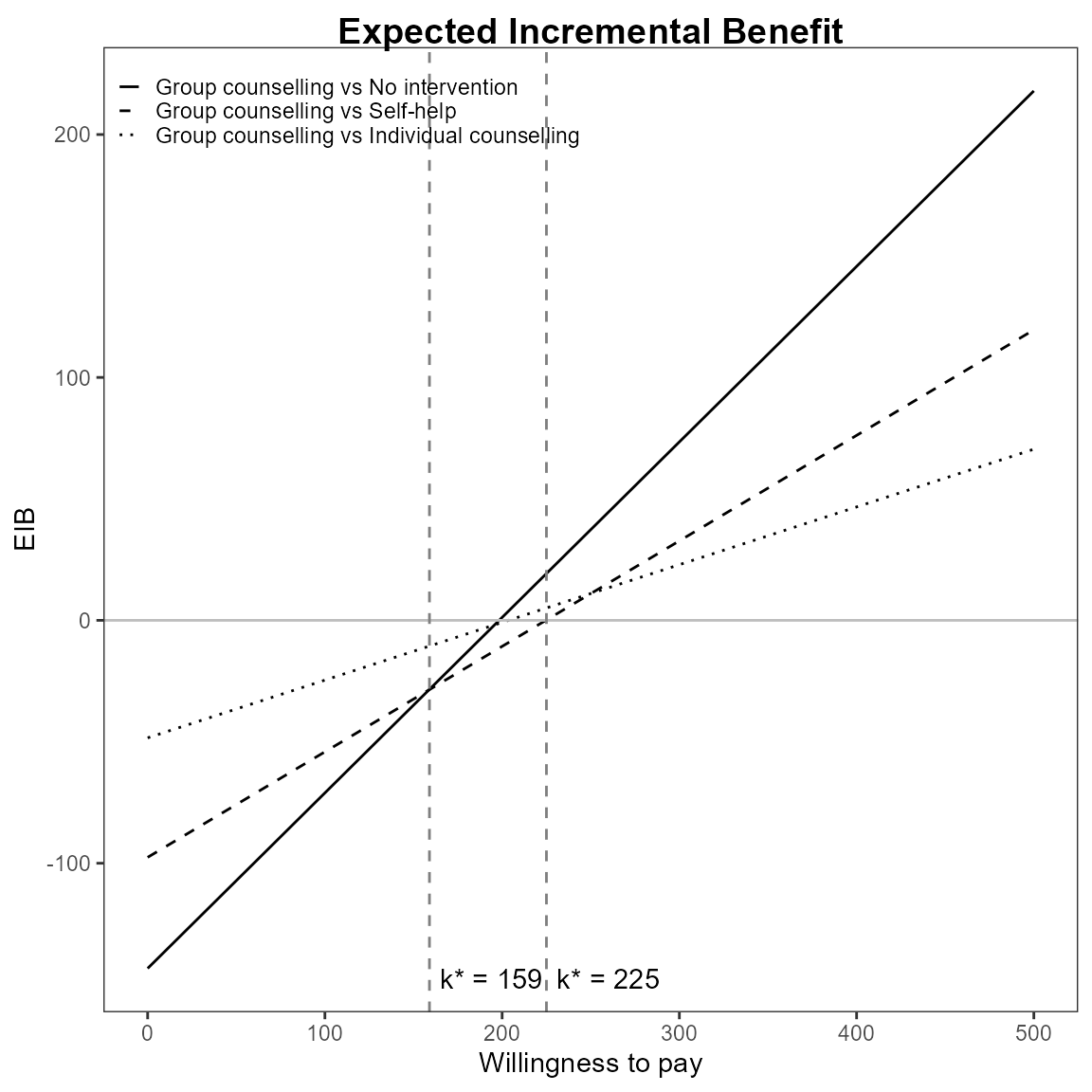eib.plot(he, graph = "ggplot2", pos = c(1, 0))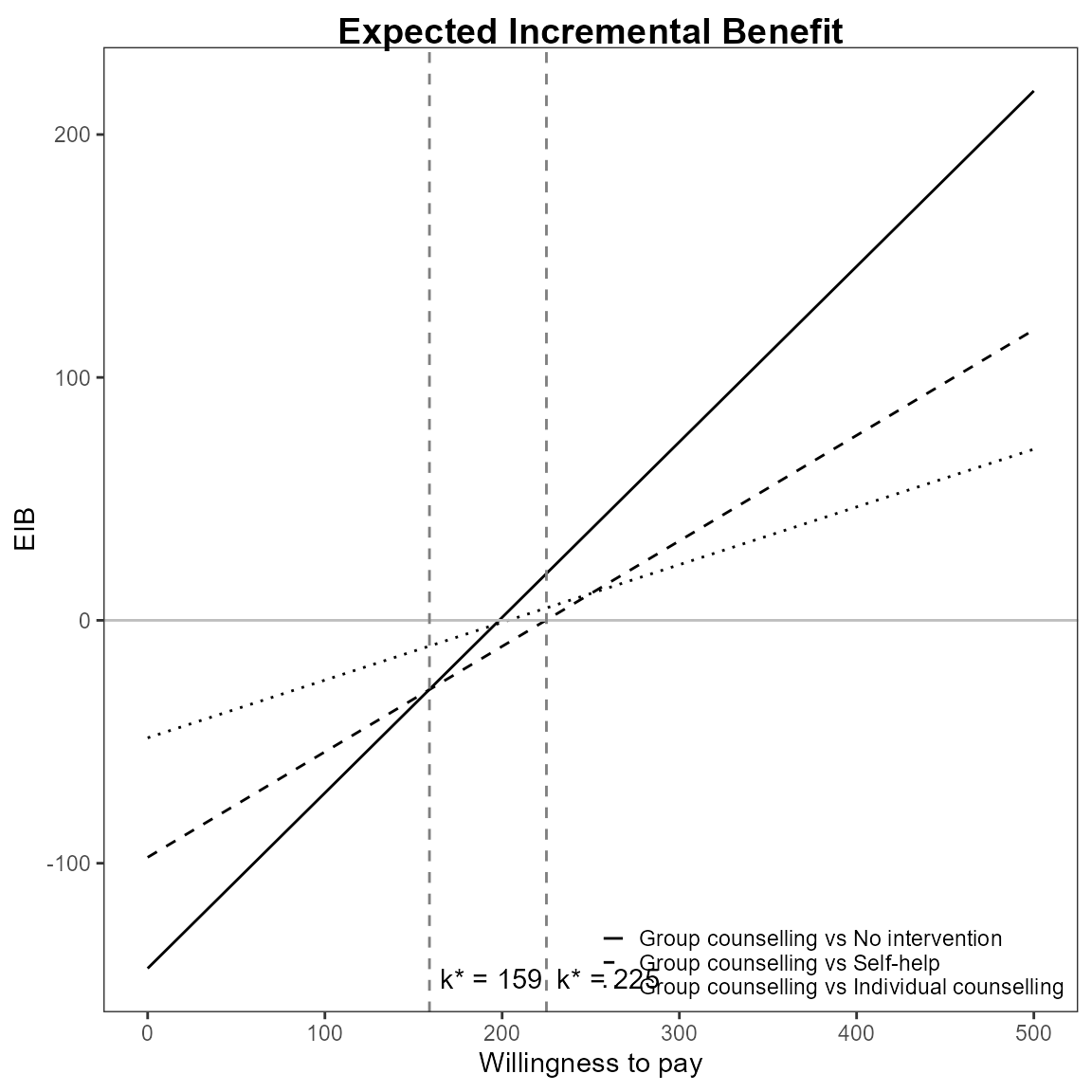eib.plot(he, graph = "ggplot2", pos = c(1, 1))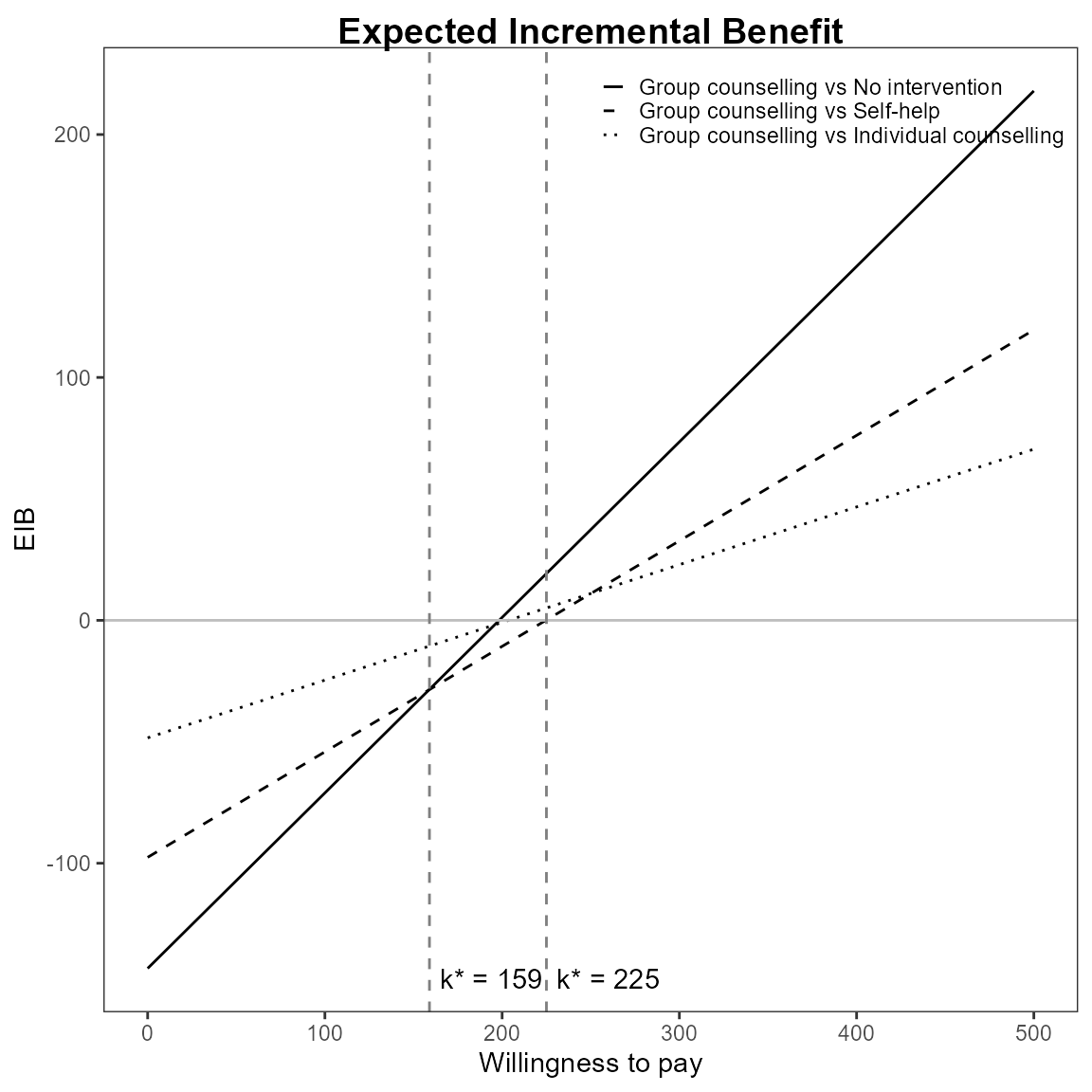Define colour palette for different colour for each EIB line.

mypalette <- RColorBrewer::brewer.pal(3, "Accent")

eib.plot(he,
graph = "base",
line = list(color = mypalette))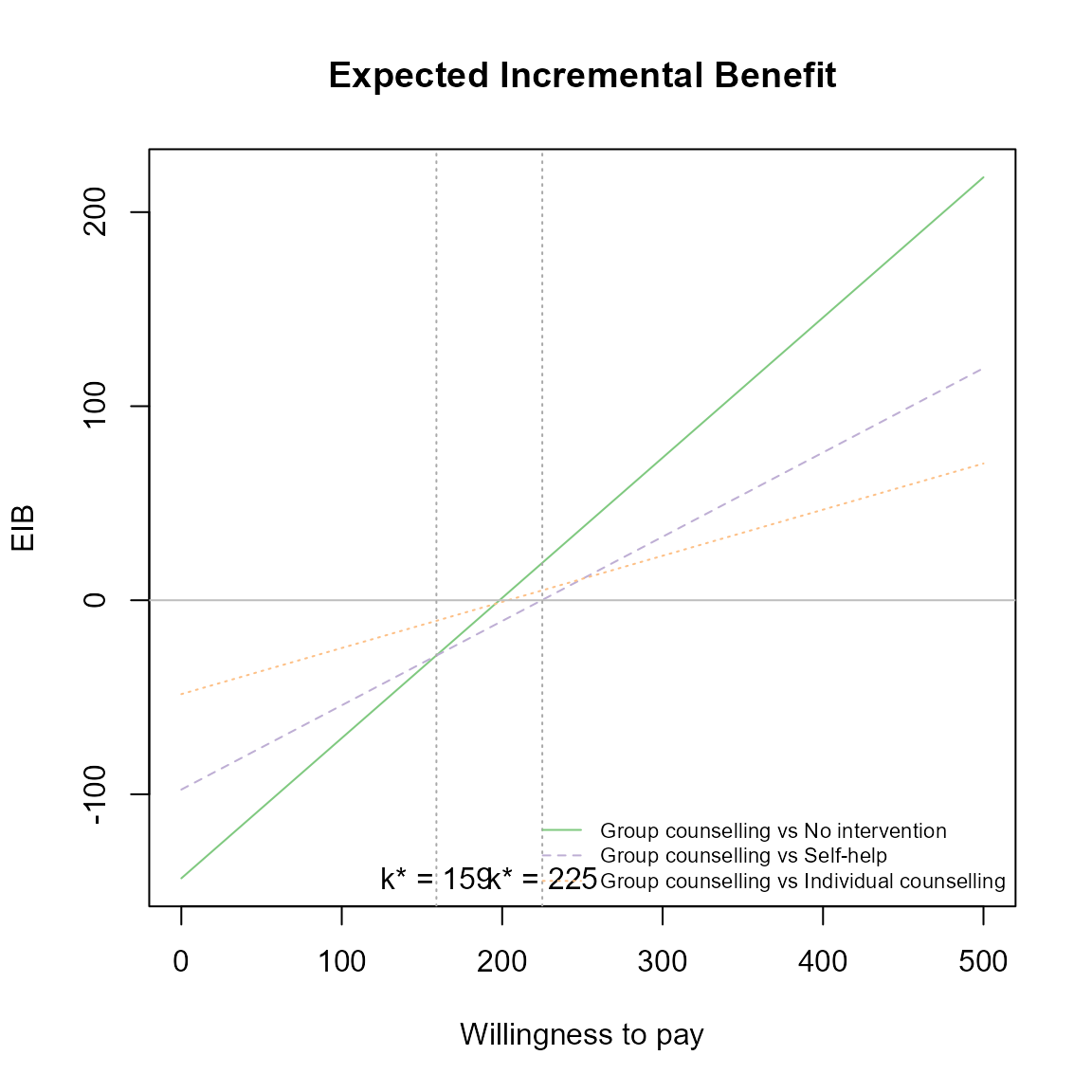eib.plot(he,
graph = "ggplot2",
line = list(color = mypalette))